Algebra II : Calculating Probability

Example Questions

← Previous 1 3 4 5

Example Question #1 : Calculating Probability

A biased coin is tossed. The probability of landing heads is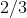, and the probability of landing tails is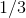.  What is the probability of tossing three tails in a row, followed by one head?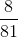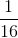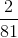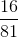Explanation:

Each coin toss is an independent event, meaning the outcome of one toss does not affect the outcome of the other coin tosses.  To find the overall probability, multiply the probabilities of each toss together: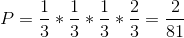Example Question #2 : Calculating Probability

A bag of marbles contains 4 gold and 6 blue marbles. What is the probability that you will draw first a blue marble then a gold marble? Assume the first marble you draw is not placed back in the bag.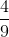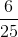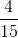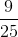Explanation:

Selecting a specific sequence of marbles from the bag without replacement is a dependent or conditional probabiliy. If you successfully draw a blue marble, it affects the chances of subsequently drawing a gold marble.

In the notation of probability we can call the probability of drawing a blue marble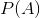and the probability of drawing a gold marble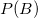.

If we replaced the first marble we drew, we would simply multiplytimes, but since we aren't we want to know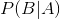or, the probability of B, given A.

So the probability of A and B is equal to: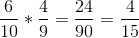In words, you have a six in ten chance of drawing a blue marble. If you successfully do that, you then have a four in nine chance of drawing a gold marble. Multiply those odds together, and reduce the fraction and you get a four in fifteen probability.

Example Question #1 : Calculating Probability

50 high school seniors were asked about their plans following graduation. 20 planned to attend an in state college. 12 planned to attend an out of state college. 8 did not plan to attend college and 10 were undecided.

What percent of this group of seniors is not planning to attend an in state college?

30%

40%

60%

20%

16%

60%

Explanation:

First, calculate the number of students not planning on attending an in state college.

Either subtract those planning on attending an in state college from the total...

50 - 20 = 30

...or add up all the other categories.

12 + 8 + 10 = 30

Then divide by the total number of students.

30/50 = 60%

Example Question #4 : Calculating Probability

If a coin is tossed in the air and a 6-sided dice is rolled, what is the probability of getting a tail on the coin and a 3 on the die?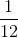Explanation: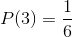Multiply these two probabilities together: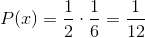Therefore the probability of flipping a tail on a coin and rolling a 3 is.

Example Question #5 : Calculating Probability

Suppose a loaded coin lands on headstimes.

What is the probability that the coin will land HTTH?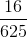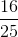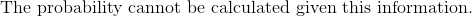Explanation:

The probability of flipping the coin in this particular order is calculated by multiplying the probabilities of each turn by each other. Because we have a "loaded" coin the probabilities are as follows: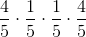.

Then simplify: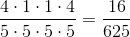.

This fraction is in its simplest form.

Example Question #1 : Probability

What is the probability of drawing 2 aces from a standard deck of playing cards when the first card is not replaced?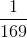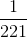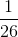Explanation:

There are 4 aces in a deck of 52 playing cards.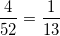After 1 ace is removed, there are 3 aces and 51 cards remaining: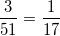To determined the probability of both events, multiply the probability of drawing each card: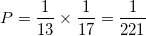Example Question #7 : Calculating Probability

In a sample of 100 high school students, 65 students played an organized sport. 48 students have a dog as a pet. What is the probability that a randomly selected student has a dog and plays a sport?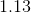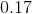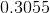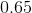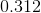Explanation:

Playing a sport, which we can call P(A), and owning a dog, which we can call P(B), are traits that we can assume are independent. The probability of one does not affect the probability of the other.

So, P(A and B) = P(A) * P(B)

Fill in the probabilities given into this equation.

P(A and B) = (0.65) * (0.48) = 0.312 = 31.2%

Example Question #8 : Calculating Probability

In a sample of cars at a parking garage, the probability of a randomly selected car being blue was found to be 0.22. The probability of a car being blue OR green was found to be 0.33. What is the probability of car from this garage being green? (Assume a car can be only one color.)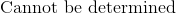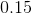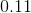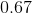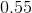Explanation:

The probability of finding either a blue car (A) or a green car (B) is written
P(A or B).

This type of probability can be found using the equation:

P(A or B) = P(A) + P(B) - P(A and B)

We need to solve this equation for P(B).

Two of the terms are given in the prompt:

P(A or B) = 0.33

P(A) = 0.22

The third, P(A and B) can be inferred. Since a car can be only one color,
P(A and B) = 0

Thus,

0.33 = 0.22 + P(B) - 0

P(B) = 0.11

Example Question #9 : Calculating Probability

In a standard deck of card, what is the probality of drawing either a face card or a red card?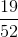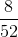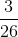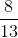Explanation:

The probability of drawing a face card P(A) or a red card P(B) can be written as P(A or B), and be calculated using:

P(A or B) = P(A) + P(B) - P(A and B)

The first two terms on the right side of the equation are fairly straightforward. Since we'll be adding and subtracting fractions, I will keep the denominator the same and won't reduce the fractions until the end.

P(A) = 12/52

P(B) = 26/52 = 1/2

The third, P(A and B), can be calculated using:

P(A and B) = P(A) * P(B)

P(A and B) = 12/52 * 1/2 = 12/104 = 6/52

(Conversely, think there are 12 face cards in a deck of 52, half of which  are red.)

Back to our original equation!

P(A or B) = 12/52 + 26/52 - 6/52

= 32/52 = 8/13

Example Question #10 : Calculating Probability

A restaurant serves apple pie and cherry pie.  On average, 60% of the customers choose apple pie.  If the next 4 customers order pie, what is the probability 3 of them order the cherry pie?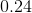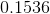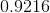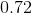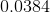Explanation:

Binomial probability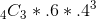← Previous 1 3 4 5

All Algebra II Resources